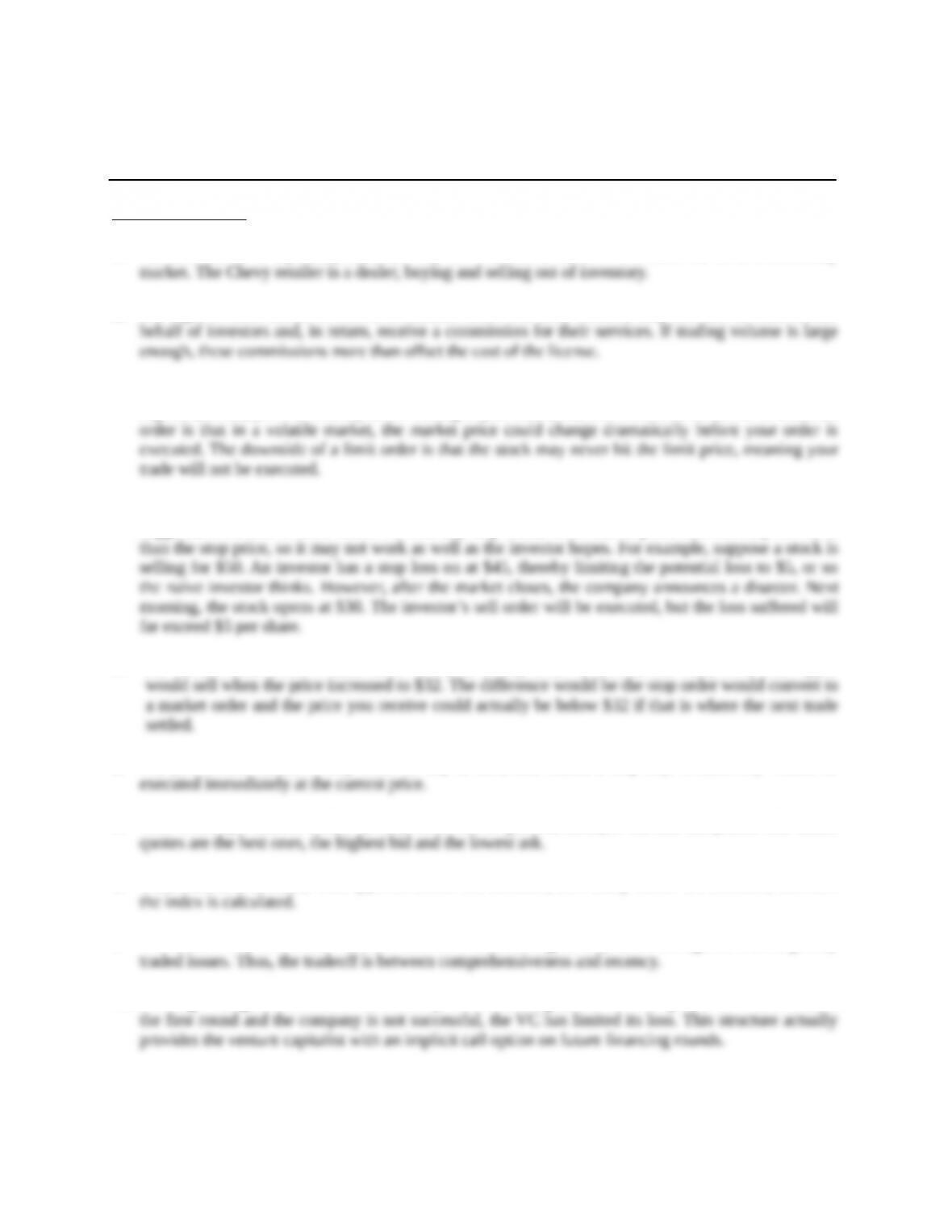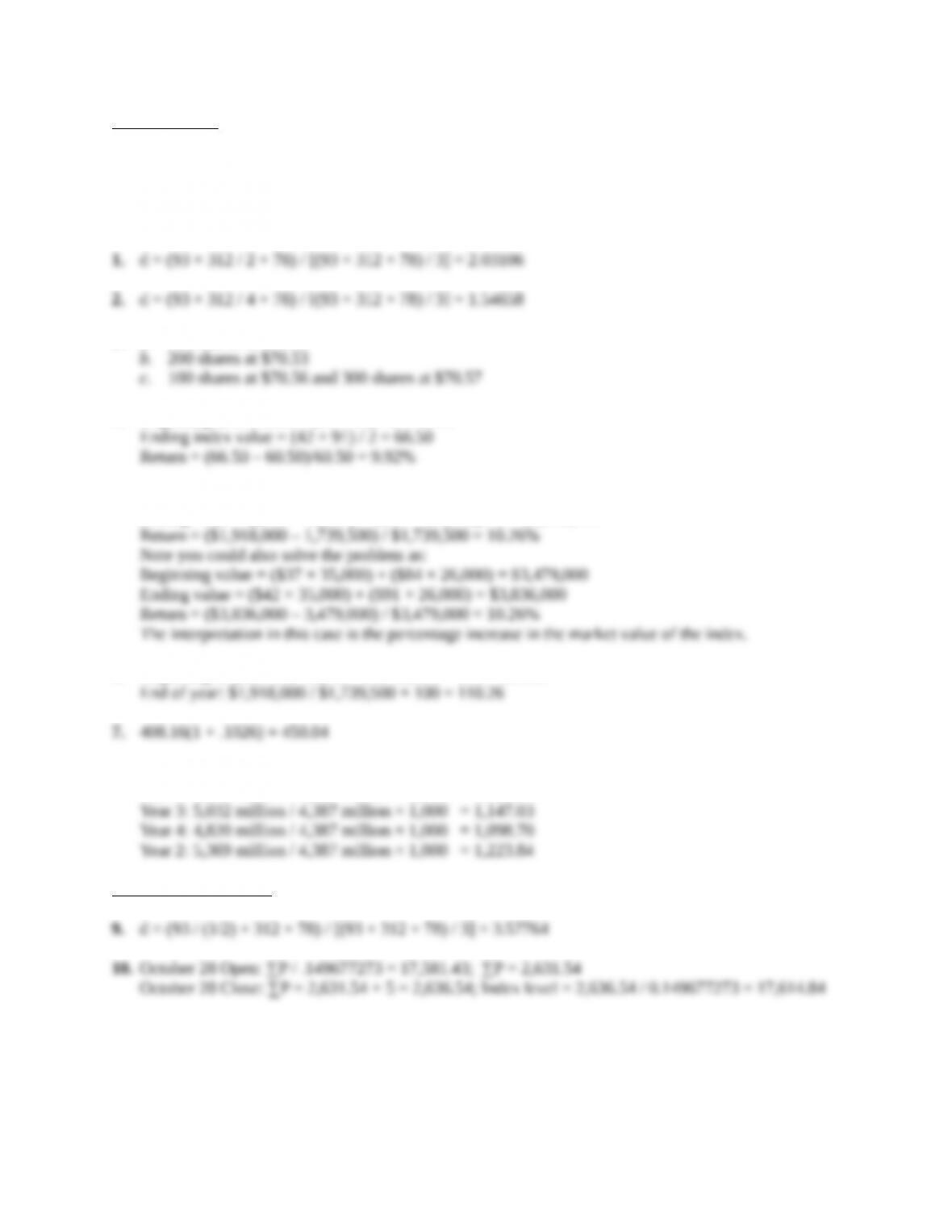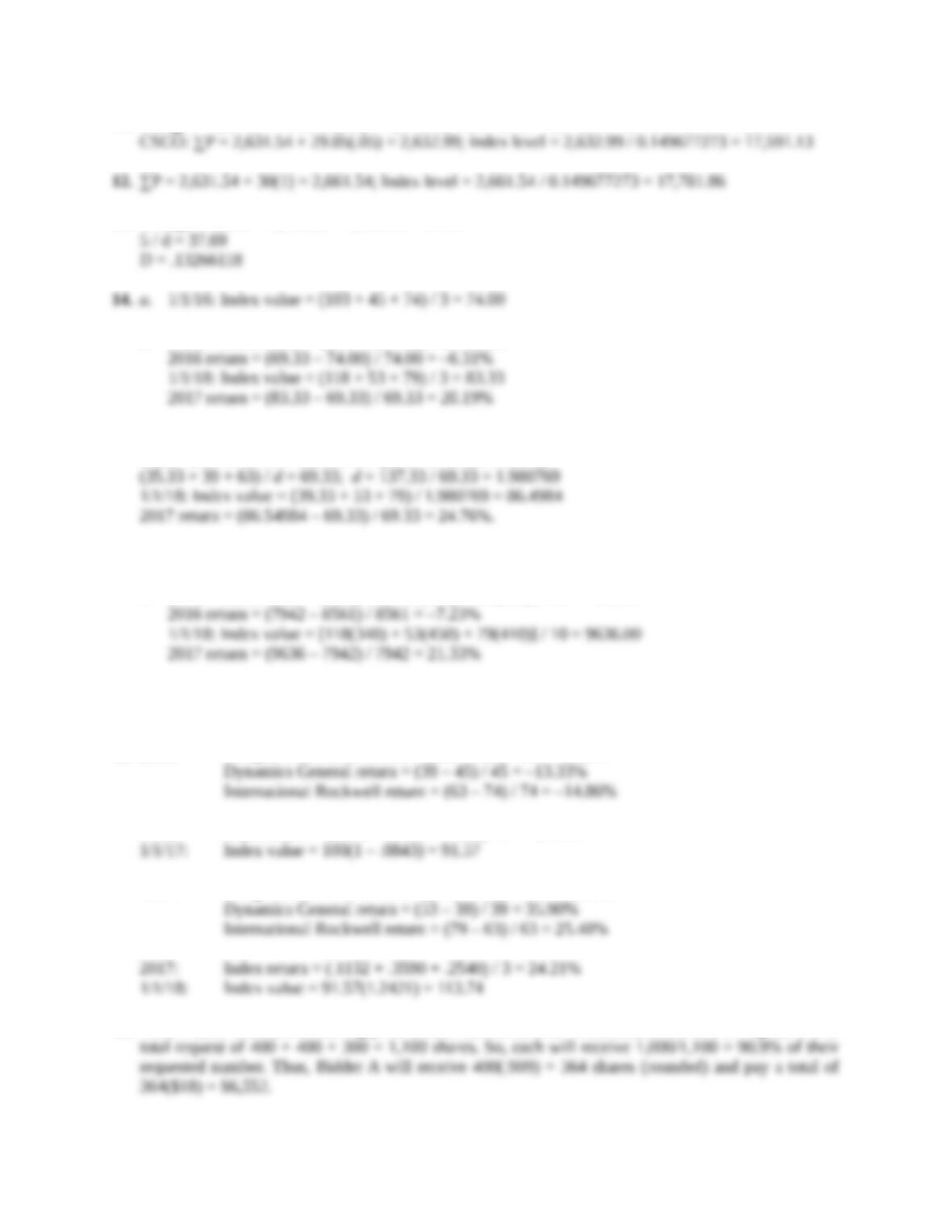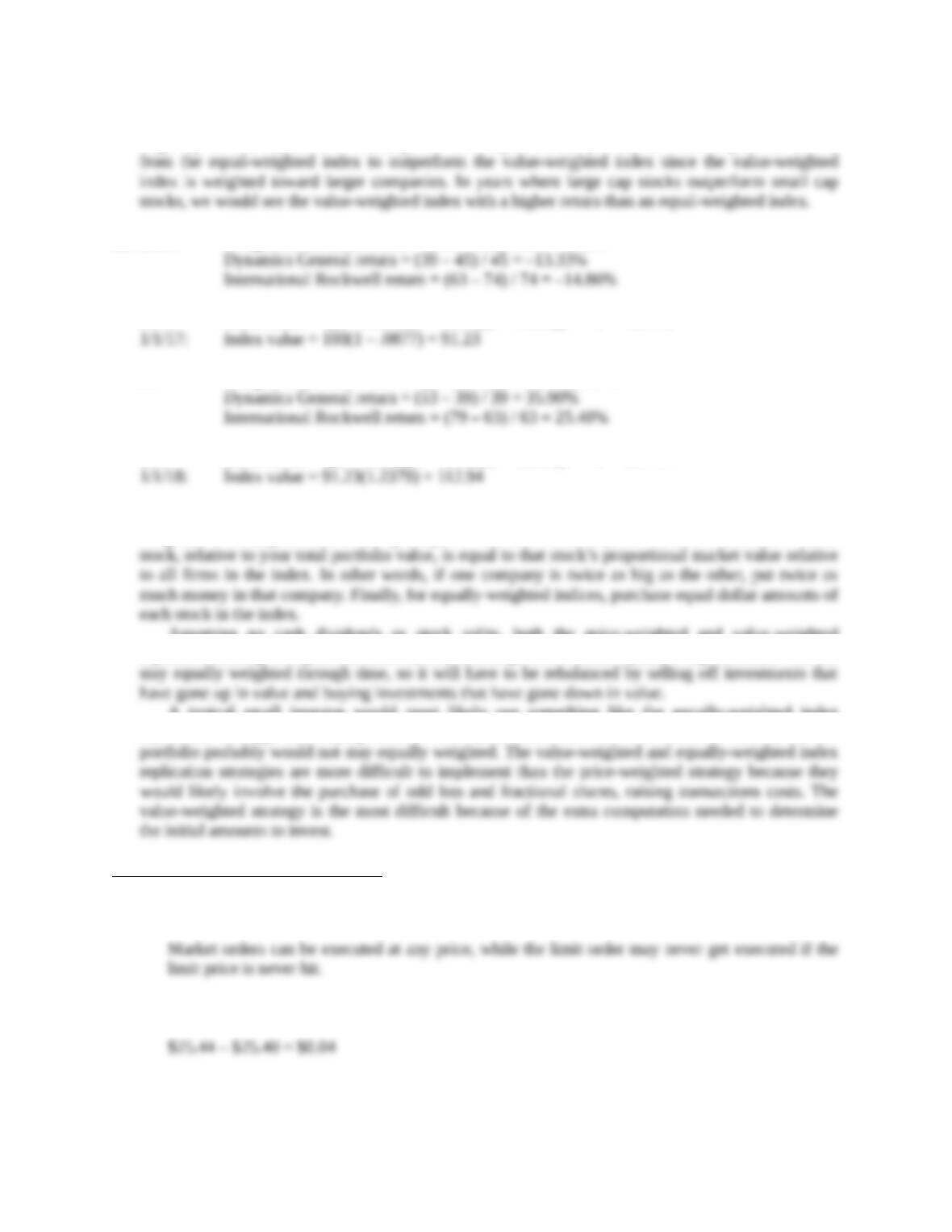Type
Solution Manual
Book Title
Fundamentals of Investments: Valuation and Management 8th Edition
ISBN 13
978-1259720697

### 978-1259720697 Chapter 5 Solution Manual

January 2, 2020Chapter 5
The Stock Market
Concept Questions
1. The new car lot is a primary market; every new car sold is an IPO. The used car lot is a secondary
2. The right to trade on the NYSE is a valuable asset. For floor brokers, they are able to trade on the
3. A market order is an order to execute the trade at the current market price. A limit order specifies the
highest (lowest) price at which you are willing to purchase (sell) the stock. The downside of a market
4. A stop-loss order is an order to sell at market if the price declines to the stop price. As the name
suggests, it is a tool to limit losses. As with any stop order, however, the price received may be worse
5. You could submit either a limit order to sell or a stop order to sell. In this case, both order types
6. No, you should submit a stop order to buy at \$17, also called a stop buy. A limit buy would be
7. With a multiple market maker system, there are, in general, multiple bid and ask prices. The inside
8. What market is covered; what types of stocks are included; how many stocks are included; and how
9. The issue is index staleness. As more stocks are added, we generally start moving into less frequently
10. Funding in stages reduces the risk faced by the venture capitalist. For example, if the VC invests in
Education.Core Questions
NOTE: All end of chapter problems were solved using a spreadsheet. Many problems require multiple
steps. Due to space and readability constraints, when these intermediate steps are included in this
solutions manual, rounding may appear to have occurred. However, the final answer for each problem is
found without rounding during any step in the problem.
3. a. 100 shares at \$70.56
4. Beginning index value = (37 + 84) / 2 = 60.50
5. Beginning value = [(\$37 × 35,000) + (\$84 × 26,000)] / 2 = \$1,739,500
Ending value = [(\$42 × 35,000) + (\$91 × 26,000)] / 2 = \$1,918,000
6. Beginning of year: \$1,739,500 / \$1,739,500 × 100 = 100.00
8. Year 1: 4,387 million / 4,387 million × 1,000 = 1,000.00
Year 2: 4,671 million / 4,387 million × 1,000 = 1,064.74
Intermediate Questions
Education.11. GS: ∑P = 2,631.54 + 186.31(.05) = 2,640.86; Index level = 2,640.86 / 0.149677273 = 17,643.67
13. Change in index = 8,503.21 – 8,465.52 = 37.69
b. 1/1/17: Index value = (106 + 39 + 63) / 3 = 69.33
15. Share price after the stock split is \$35.33 (\$106 × (1/3)).
Index value on 1/1/14 without the split is 69.33 (see above).
16. a. 1/1/16: Index value = [103(340) + 45(450) + 74(410)] / 10 = 8561.00
b. 1/1/17: Index value = [106(340) + 39(450) + 63(410)] / 10 = 7942.00
17. The index values and returns will be unchanged; the stock split changes the share price, but not the
total value of the firm.
18. 2016: Douglas McDonnell return = (106 – 103) / 103 = 2.91%
2016: Index return = (.0291 – .1333 – .1486) / 3 = –8.43%
2017: Douglas McDonnell return = (118 – 106) / 106 = 11.32%
19. To sell 1,000 shares, the clearing price would need to be \$18. Three bidders bid \$18 or higher, with a
Education.20. Looking back at Chapter 1, you can see that there are years in which small cap stocks outperform
large cap stocks. In years with better performance by small companies, we would expect the returns
21. 2016: Douglas McDonnell return = (106 – 103) / 103 = 2.91%
2016: Index return = [(1 + .0291)(1 – .1333)(1 – .1486)]1/3 – 1 = –8.77%
2017: Douglas McDonnell return = (118 – 106) / 106 = 11.32%
2017: Index return = [(1 + .1132)(1 + .3590)(1 + .2540)]1/3 – 1 = 23.79%
22. For price-weighted indices, purchase an equal number of shares for each firm in the index. For value-
weighted indices, purchase shares (perhaps in fractional amounts) so that the investment in each
Assuming no cash dividends or stock splits, both the price-weighted and value-weighted
replication strategies require no additional rebalancing. However, an equally weighted index will not
A typical small investor would most likely use something like the equally-weighted index
replication strategy, i.e., buying more-or-less equal dollar amounts of a basket of stocks, but the
CFA Exam Review by Kaplan Schweser
1. a
2. c
3. b